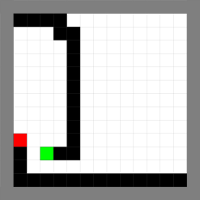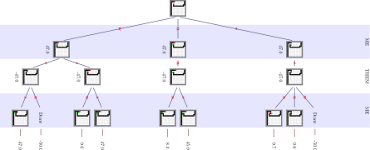# A simple Tron bot

The University of Waterloo Computer Science Club runs an interesting AI challenge sponsored by Google. The aim of the challenge is to write a computer program that plays Tron.

Tron is a two-player game where each player controls a snake trapped inside a maze. When a player moves her snake, it grows leaving a solid wall behind. The last player to crash on a wall or on a snake's trail wins. If the two players crash simultaneously, it is a draw.

I was attracted by the simplicity of the rules, so I decided to write a bot.

## Minimax

The bot is based on the well known minimax algorithm for zero-sum games.

The idea of minimax is to consider all the possibles outcomes given a starting state of the board, until a given depth. To achieve this, it starts by considering our bot's admissible moves. Each move considered creates a possible future, that is represented as a node in a search tree. For each future (or node) we then consider each one of the opponent's possible moves. We iterate this process until the maximum depth is reached.

As an example, we are going to consider the following game position, our bot is represented in green, the opponent in red:If we apply minimax search to this position with a depth of 3, we produce the search tree to the right. We start with the initial position at the root of the tree. It is our turn to play, moving to the east would kill us, so we are left with three valid moves: west, south and north, that produce the three boards in the first blue area.

Then our opponent considers her available moves for each one of the boards. Depending on her move, and on our next move, we arrive to one of the 14 leaf positions.

## Evaluation

Now that we have outlined all the possible futures after 3 turns, we would like to chose the move that drives us to the most advantageous position (possibly a winning position).

We start by evaluating each leaf position. When the position is an end game position the evaluation is simple. A position is graded:

• -100 points if the opponent has won
• 100 points if we have won
• -50 points for a draw

When the position is not an end game position, we have to evaluate whether our position is a strong one. We consider two scenarios:

• When our bot and the opponent's are separated by a wall, we evaluate the free space that each bot has. Strong positions are the ones where our bot has more free space.

• When we are not separated, we prefer the positions where the two bots are close. We choose this strategy because our bot is better at close combat. Indeed when the bots are very far apart, the exploration depth needed to consider attacks on the opponent is too deep for our minimax algorithm. On the other hand when the bots are close, the minimax algorithm can find clever attacks to entrap our enemy!

## Propagating the evaluation

Now that we can grade each one of the leaf positions, we want to propagate these evaluations back to the first level of the tree, so we can decide what the best available move in the initial position is.

In our turn we make the best possible move. Therefore the evaluation of a board in a white region (just before our turn), is the maximum of the evaluation of its children. Similarly, when our adversary plays, we predict that she will make the worst possible move (from our point of view). Therefore the evaluation of a board in a blue region, is the minimum of the evaluation of its children.

For example consider the leaf positions s-e-w and s-e-e:

• s-e-w is a very bad position, we are trapped with little space around us, so its grade is -75.2

• s-e-e is better, the opponent is trapped, so its grade is 47.0

Because both positions are the result of one of our moves, to compute the grade of s-e, we take the maximum of 75.2 and 47.0.

By iterating this process, we are able to grade the positions at level one. Our bot will move to the position with the greater grade, in this case w , which entraps the enemy.

## Speed considerations

The challenge has an additional rule: a bot is disqualified if it takes more than one second to compute its move. It is thus very important to be efficient in the second that is alloted to us. We apply different optimizations to the basic minimax algorithm:

### Alpha-Beta Pruning

This is a cutting technique which discards suboptimal branches of the minimax search. In our example, when we explore the branch s-n we grade it 45.0. So we know that the branch s will be graded at maximum 45.0, since the opponent chooses the worst possible outcome. We also know that the branch w is graded 47.0 (more than 45.0), therefore branch s will never be taken. By this line of reasoning, branch s-e can be cut.

Below is the alpha-beta pruned search tree of the above example, as you see alpha-beta can cut many branches, giving us more time to explore the worthy ones.### Iterative Deepening

The efficiency of alpha-beta pruning depends on the order in which the positions are explored. The idea behind iterative deepening is to first explore depth 1, then order the moves according to their grades. Then explore depth 2, in the order found during the previous exploration, and so on.

Iterative deepening repeats some of its work since for each exploration it has to start back at depth 1. But the gains that it provides by correctly ordering the nodes outweight the cost of the repetition.

Iterative deepening is also useful, since it allows us to refine the solution gradually. That means that when we are exploring depth d, we already have a good solution for depth (d-1). What we do with our bot, is keep track of the time remaining to return a solution. When we are almost out of time, we stop iterative deepening and return the last best solution.